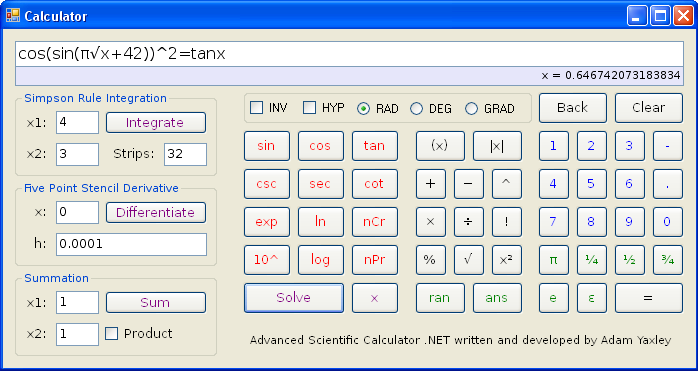# Background

I have a passion for mathematics. This passion was ignited during my A levels (last year of high school), mainly because of the superb teachers, for which I am grateful. My chosen A level subjects were were Maths, Further Maths and Physics, so you can imagine how geeky I was. Being a programmer, I could see how the topics that I was studying could be automated. Utilising C++ and diving into .NET, I created a calculator application, that could essentially check my homework for me.

For example, have you ever wondered what x was in the following formula?

cos(sin(π√x + 42))² = tanx

Of course x = 0.646742073183834, but how would you know without using my miraculous calculator?# Features

• Integration approximation
• Differentiation approximation
• Iterative equation solving
• Summation functionality
• Trigonometric functions
• Logarithmic functions
• Correct operator precendence rules
• Human friendly input (can write 5x to mean 5 multiplied by x)
• Highlight an expression and click on the (x) or |x| button to wrap it in parenthesis.
• Support for Degrees, Radians, and Gradians.